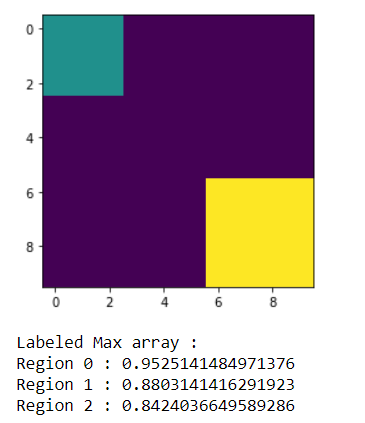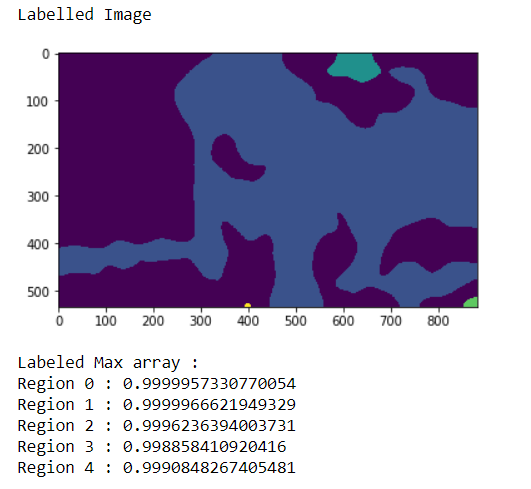Skip to content
Related Articles
Mahotas – Getting Labeled Max Array
• Last Updated : 04 May, 2021

In this article we will see how we can obtain the labeled max array of the in mahotas. Labeled images are integer images where the values correspond to different regions. I.e., region 1 is all of the pixels which have value 1, region two is the pixels with value 2, and so on. By convention, region 0 is the background and often handled differently. We can create a labelled region with the help of mahotas.label method.
We can get the size of each region with the help of mahotas.label_size method, this size is measured simply as the number of pixels in each region. We can instead measure the total weight in each area.
In order to do this we will use mahotas.labeled.labeled_max method

Syntax : mahotas.labeled.labeled_max(array, labeled_img)
Argument : It takes two numpy.ndarray object as argument i.e random array of region shape and labeled region
Return : It returns ndarray of numpy.float64 values

Example 1:

## Python3

 `# importing required libraries``import` `mahotas``import` `numpy as np``from` `pylab ``import` `imshow, show`` ` `# creating region``# numpy.ndarray``regions ``=` `np.zeros((``10``, ``10``), ``bool``)`` ` `# setting 1 value to the region``regions[:``3``, :``3``] ``=` `1``regions[``6``:, ``6``:] ``=` `1`` ` `# getting labeled function``labeled, nr_objects ``=` `mahotas.label(regions)`` ` `# showing the image with interpolation = 'nearest'``imshow(labeled, interpolation ``=``'nearest'``)``show()`` ` `     ` `# random agaary of region shapes   ``array ``=` `np.random.random_sample(regions.shape)`` ` `# getting labeld max array``l_array ``=` `mahotas.labeled.labeled_max(array, labeled)` `print``(``"Labeled Max array :"``)``# printing the  values``for` `i ``in` `range``(``len``(l_array)):``    ``print``(``"Region "` `+` `str``(i) ``+` `" : "` `+` `str``(l_array[i]))`

Output :```Labeled Max array :
Region 0 : 0.9980513142322492
Region 1 : 0.624390200202312
Region 2 : 0.9210927640926101```

Example 2:

## Python3

 `# importing required libraries``import` `numpy as np``import` `mahotas``from` `pylab ``import` `imshow, show`` ` `# loading image``img ``=` `mahotas.imread(``'dog_image.png'``)``   ` `# filtering the image``img ``=` `img[:, :, ``0``]``    ` `# setting gaussian filter``gaussian ``=` `mahotas.gaussian_filter(img, ``15``)`` ` `# setting threshold value``gaussian ``=` `(gaussian > gaussian.mean())`` ` `# creating a labeled image``labeled, n_nucleus ``=` `mahotas.label(gaussian)``  ` `print``(``"Labelled Image"``)``# showing the gaussian filter``imshow(labeled)``show()`` `  `# random agary of region shapes   ``array ``=` `np.random.random_sample(labeled.shape)` `# getting labeld max array``l_array ``=` `mahotas.labeled.labeled_max(array, labeled)` `print``(``"Labeled Max array :"``)``# printing the  values``for` `i ``in` `range``(``len``(l_array)):``    ``print``(``"Region "` `+` `str``(i) ``+` `" : "` `+` `str``(l_array[i]))`

Output :```Labeled Max array :
Region 0 : 0.9999995478951564
Region 1 : 0.9999944289534851
Region 2 : 0.999718434740755
Region 3 : 0.9996236088210476
Region 4 : 0.9861670123032187```

Attention geek! Strengthen your foundations with the Python Programming Foundation Course and learn the basics.

To begin with, your interview preparations Enhance your Data Structures concepts with the Python DS Course. And to begin with your Machine Learning Journey, join the Machine Learning – Basic Level Course

My Personal Notes arrow_drop_up